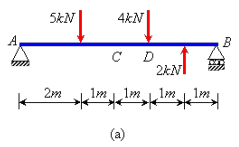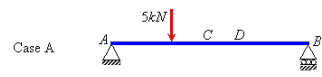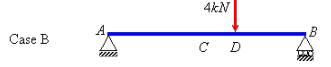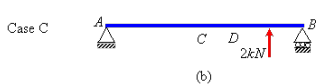# Use of Influence Lines Civil Engineering (CE) Notes | EduRev

## Civil Engineering (CE) : Use of Influence Lines Civil Engineering (CE) Notes | EduRev

The document Use of Influence Lines Civil Engineering (CE) Notes | EduRev is a part of the Civil Engineering (CE) Course Structural Analysis I.
All you need of Civil Engineering (CE) at this link: Civil Engineering (CE)

Use of Influence Lines

In this section, we will illustrate the use of influence lines through the influence lines that we have obtained in Section 6.2. Let us consider a general case of loading on the simply supported beam (Figure 6.4a) and use the influence lines to find out the response parameters (RA,Vc and MD) for their loading. We can consider this loading as the sum of three different loading conditions, (A), (B) and (C) (Figure 6.4b), each containing only one externally applied force.For loading case (A), we can find out the response parameters using the three influence lines. Ordinate of an influence line gives the response for a unit load acting at a certain point.

Therefore, we can multiply this ordinate by the magnitude of the force to get the response due to the real force at that point. Thus

RA = 5kN x (ordinate of the IL of RA at X = 2m) = 5(1-2/6) = 3.33kN

Vc = 5kN x (ordinate of the IL of Vc at X = 2m) = 5(2/6) = 1.67 kN

MD = 5kN x (ordinate of the IL of MD at x = 2m) = 5(2/3) = 3.33 kNm

RA = 4kN x (ordinate of the IL of RA at x = 4m) = 4(1-4/6) = 1.33kN

Vc = 4kN x (ordinate of the IL of Vc at x = 4m)  = 4(4/6-1) = 1.33kN

MD = 4kN x (ordinate of the IL of Mat  x = 4m)  = 4(2 x 6/9) 5.33 kNm

And for case (C),

RA = -2kN x (ordinate of the IL of RA at x = 5m) = -2(1-5/6) = -0.33kN

Vc = -2kN x (ordinate of the IL of Vc at x = 5m)  = -2(5/6-1) = 0.33kN

MD= -2kN x (ordinate of the IL of MD at x = 5m)  = -2(2 x 6/3-2 x 5/3) = -1.33kNm

By the theory of superposition, we can add forces for each individual case to find the response parameters for the original loading case (Figure 6.4a). Thus, the response parameters in the beam AB are:

RA = (3.33 + 1.33 - 0.33)kN=4.33kN

Vc = (1.66 - 1.33 + 0.33)kN=0.67 kN

MD = (3.33 + 5.33 - 1.33)kN=7.33 kNm

One should remember that the method of superposition is valid only for linear elastic cases with small displacements only. So, prior to using influence lines in this way it is necessary to check that these conditions are satisfied.

It may seem that we can solve for these forces under the specified load case using equilibrium equations directly, and influence lines are not necessary. However, there may be requirement for obtaining these responses for multiple and more complex loading cases. For example, if we need to analyse for ten loading cases, it will be quicker to find only three influence lines and not solve for ten equilibrium cases.

The most important use of influence line is finding out the location of a load for which certain response will have a maximum value. For example, we may need to find the location of a moving load (say a gantry) on a beam (say a gantry girder) for which we get the maximum bending moment at a certain point. We can consider bending moment at point D of Example 6.3, where the beam AB becomes our gantry girder.Looking at the influence line of MD,one can say that MD will reach its maximum value when the load is at point D.Influence lines can be used not only for concentrated forces, but for distributed forces as well, which is discussed in the next section.

Offer running on EduRev: Apply code STAYHOME200 to get INR 200 off on our premium plan EduRev Infinity!

## Structural Analysis I

23 videos|33 docs|18 tests

,

,

,

,

,

,

,

,

,

,

,

,

,

,

,

,

,

,

,

,

,

;